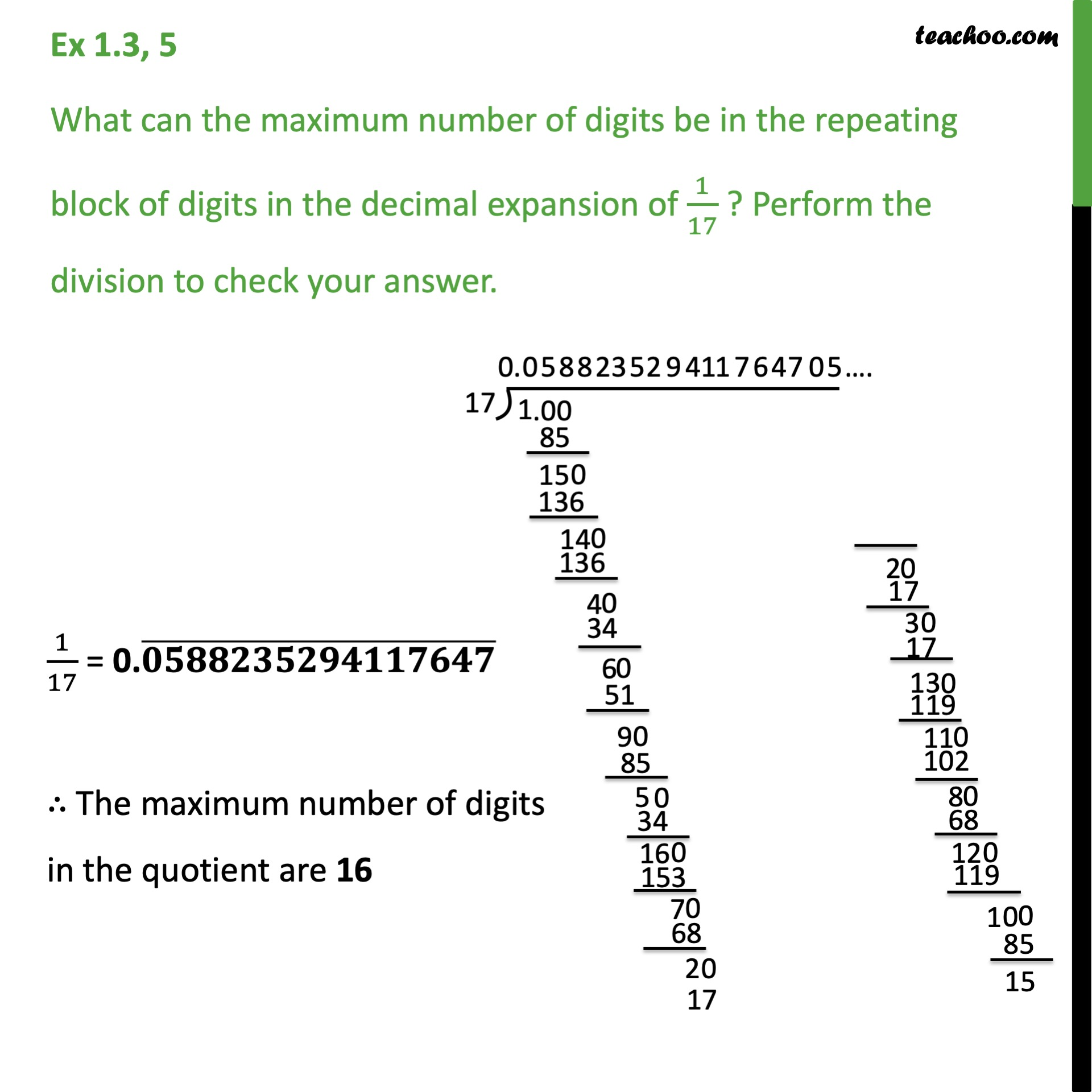1. Chapter 1 Class 9 Number Systems (Term 1)
2. Concept wise
3. Finding decimal expansion

Transcript

Ex 1.3, 5 What can the maximum number of digits be in the repeating block of digits in the decimal expansion of 1/17 ? Perform the division to check your answer. Here, we perform division. Dividing 1 by 17 1/17 = 0.05882352954117647 bar Therfore, Maximum number of digits in the quotient while computing 1/17 are 16